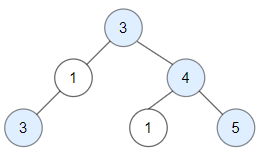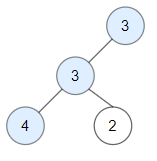Formatted question description: https://leetcode.ca/all/1448.html

# 1448. Count Good Nodes in Binary Tree (Medium)

Given a binary tree root, a node X in the tree is named good if in the path from root to X there are no nodes with a value greater than X.

Return the number of good nodes in the binary tree.

Example 1:Input: root = [3,1,4,3,null,1,5]
Output: 4
Explanation: Nodes in blue are good.
Root Node (3) is always a good node.
Node 4 -> (3,4) is the maximum value in the path starting from the root.
Node 5 -> (3,4,5) is the maximum value in the path
Node 3 -> (3,1,3) is the maximum value in the path.

Example 2:Input: root = [3,3,null,4,2]
Output: 3
Explanation: Node 2 -> (3, 3, 2) is not good, because "3" is higher than it.

Example 3:

Input: root = 
Output: 1
Explanation: Root is considered as good.

Constraints:

• The number of nodes in the binary tree is in the range [1, 10^5].
• Each node's value is between [-10^4, 10^4].

Related Topics:
Tree, Depth-first Search

## Solution 1. Pre-order traversal

We can simply use pre-order traversal.

When visiting a node p, we pass in the maximum value maxVal we’ve seen from the root thus far.

If p->val >= maxVal, we increment the answer.

Then we update maxVal = max(maxVal, root->val), and continue to visit p’s child nodes.

// OJ: https://leetcode.com/problems/count-good-nodes-in-binary-tree/

// Time: O(N)
// Space: O(H)
class Solution {
int ans = 0;
void dfs(TreeNode *root, int maxVal) {
if (!root) return;
if (root->val >= maxVal) ++ans;
maxVal = max(maxVal, root->val);
dfs(root->left, maxVal);
dfs(root->right, maxVal);
}
public:
int goodNodes(TreeNode* root) {
dfs(root, INT_MIN);
return ans;
}
};


Java

/**
* Definition for a binary tree node.
* public class TreeNode {
*     int val;
*     TreeNode left;
*     TreeNode right;
*     TreeNode() {}
*     TreeNode(int val) { this.val = val; }
*     TreeNode(int val, TreeNode left, TreeNode right) {
*         this.val = val;
*         this.left = left;
*         this.right = right;
*     }
* }
*/
class Solution {
public int goodNodes(TreeNode root) {
if (root == null)
return 0;
int count = 1;
Queue<TreeNode> nodeQueue = new LinkedList<TreeNode>();
Queue<Integer> maxNode = new LinkedList<Integer>();
nodeQueue.offer(root);
maxNode.offer(root.val);
while (!nodeQueue.isEmpty()) {
TreeNode node = nodeQueue.poll();
int max = maxNode.poll();
TreeNode left = node.left, right = node.right;
if (left != null) {
nodeQueue.offer(left);
if (left.val >= max)
count++;
maxNode.offer(Math.max(left.val, max));
}
if (right != null) {
nodeQueue.offer(right);
if (right.val >= max)
count++;
maxNode.offer(Math.max(right.val, max));
}
}
return count;
}
}